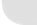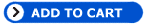Search for
Thursday 7th July 2022
 Pipe Flow Software Pipe Flow Expert Software Pipe Flow Wizard Software Pipe Flow Advisor Software Pressure Drop Theory Fluid Flow Factors Pumping the Fluid Fluid Database (PF3D) Pipe Flow 3D FAQs Convert 123 Software News & Press Releases Software Downloads Who Uses Our Software Customer Testimonials How to Buy Online Get Software License Code Software Release Dates Pipe Flow Software FAQs Technical Articles Europump Guides Contact Pipe Flow Software About Pipe Flow Software Piping Related Links Site Map Secure Online Payment# Pipe Flow 3D - Pressure Drop Theory

 This page describes in detail which factors need to be considered when calculating pressure drops through pipe systems, their effects on fluid flow and conditions at the pump inlet, and how Pipe Flow 3D can help with the calculations. Resistance to fluid flow Fluids in motion are subjected to various resistances, which are due to friction. Friction may occur between the fluid & the pipe work, but friction also occurs within the fluid as sliding between adjacent layers of fluid takes place. The friction within the fluid is due to the fluid’s viscosity.[ Now superseded by          Pipe Flow Expert ]
When fluids have a high viscosity, the speed of flow tends to be low, and resistance to flow becomes almost totally dependant on the viscosity of the fluid, this condition is known as ‘Laminar flow’.

How do you establish the pipework resistance losses?

Before the pipework losses can be established, the friction factor must be calculated. The friction factor will be dependant on the pipe size, inner roughness of the pipe, flow velocity and fluid viscosity. The flow condition, whether ‘Turbulent’ or not, will determine the method used to calculate the friction factor. The starting point must be to find the fluid’s viscosity. This will be the factor that has most effect on the pipework losses.Understanding viscosity units (dynamic viscosity)

Many terms can be used to describe a fluid's viscosity (its resistance to flow): Centipoise, Poise, Saybolt Universal (SSU), Saybolt Furol, Ford Cup No. 3, Ford Cup No.4, Redwood No.1, Degrees Engler, Zahn No.1, Zahn No.2 and Zahn No. 3 are some of the scales that have been used in the immediate past.

All of these scales have differing upper and lower values and are usually not directly related to each other. Some references may be found in text books which attempt to list equivalent values for these different methods of measuring viscosity.

Pipe Flow 3D provides a means of calculating the equivalent centistokes viscosity from some other known viscosity scales.

Kinematic viscosity and Reynold’s numbers

Dynamic viscosity must be converted to its Kinematic viscosity equivalent before the viscosity value can be used to calculate Reynold’s numbers and hence friction factors.

It is very common today to express dynamic viscosity in centipoise.
one Centipoise = 1 mPa.s or 0.001 (kg/m) x s

 The units of centipoise are: Force per unit area x Time

It is very common today to express kinematic viscosity in centistokes.
one Centistoke = 1 mm²/s or 0.000001 m²/s

 The units of centistokes are: Length² Time

 Kinematic viscosity is simply: Dynamic viscosity Mass density

Reynold’s numbers

Reynold’s numbers (Re) describe the relationship between a fluid’s velocity, the pipe size and the fluid’s kinematic viscosity.

 Reynold’s number = Fluid velocity x Internal pipe diameter Kinematic viscosity

Effect of the inner roughness of the pipe

The inner roughness of the pipe can create eddy currents. This increases the friction between the pipe wall and the fluid. The relative roughness of the inside of the pipe is used in determining the friction factor to be used.

 Relative roughness = Inside pipe roughness Inside pipe diameter

The average inner roughness of commercial pipes:

 Steel tube Copper tubing Glass tubing Polythene Flexible P.V.C. Rigid P.V.C. Cast iron tube Concrete tube 0.0460 mm 0.0015 mm 0.0001 mm 0.0010 mm 0.2000 mm 0.0050 mm 0.2600 mm2.0000 mm

Friction factor chartThe chart above shows the relationship between Reynold’s number and pipe friction.
Calculation of friction factors is dependant on the type of flow that will be encountered.

For Re numbers <2300 the fluid flow is Laminar, when Re number is >2300 the fluid flow is Turbulent.

Laminar flow (Re < 2300) f = 64/Re
Turbulent flow (Re > 2300) 1/sqrt(f) = -1.8 log [ (6.9/Re) + ((k/3.7)^1.11 ]
(where k = inner pipe roughness / inner pipe diameter)

Most commercial applications involve Turbulent flow. In these cases the inner roughness of the pipework can have a significant effect on the Friction factor.

The Relative roughness is the inner roughness divided by the internal diameter of the pipe work.
The Friction factor is found by plotting the intersection of Re and Relative roughness, and reading the friction factor on the left hand axis of the chart.

The Fluid head loss can be calculated once the friction factor is known.
The Pressure drop in pipe work can be calculated from fluid head loss, the density of fluid and the acceleration due to gravity.

Fluid head resistance can be calculated from h = f (L/d) x (v ²/2g)

where
f = friction factor
L = length of pipe work (m)
d = inner dia of pipe work (m)
v = velocity of fluid (m/s)
g = acceleration due to gravity (m/s ²)

Calculating the losses through pipe work fittings

The fluid head resistance through various pipe work fittings can be calculated when the 'K' factor of the fitting is known. Manufacturers of pipe work fittings & valves publish 'K' factors for their products.

Usually a particular type of fitting from various manufacturers have similar 'K' factors, therefore this computer program tends to use average 'K' factor values.

Fluid head loss of these fitting can be calculated from h = total 'K' x v ² / 2g

where
total 'K' = total of 'K' factors for each fitting
v = velocity of fluid (m/s)
g = acceleration due to gravity (m/s ²)

Note: If the pipework involves different pipe sizes, this calculation must be carried out separately for each pipe size, using the appropriate velocity within that pipe section.

The 'K' value of entry & exit points can be taken as 0.8 and 1.0 respectively to calculate the head loss attributable to these features.

Calculating the total pressure drop

The total fluid head resistance may be used to calculate the pressure required to overcome the resistance to fluid flow.

Pd = h x p x g / 100000

where
Pd = pressure drop (bar)
p = fluid density (kg/m3)
g = acceleration due to gravity (m/s ²)

Finally, the fluid is most likely to exit into atmospheric pressure. The difference between the pressure on the fluid surface during storage & the atmospheric pressure must be taken into account in determining the pressure drop to be overcome by the pump.

This difference in pressure may be positive (assisting fluid flow) or negative (resisting fluid flow).

Summarising the steps to be considered

Factors that affect fluid flow

Fluid flow in pipes is affected by many different factors:

• The viscosity, density, and velocity of the fluid.
• Changes in the fluid temperature will change the viscosity & density of the fluid.
• The length, inner diameter, and in the case of turbulent flow, the internal roughness of the pipe.
• The position of the supply and discharge containers relative to the pump position.
• The addition of rises & falls within the pipe layout.
• The number & types of bends in the pipe layout.
• The number & types of valves, & other fittings, in the pipe layout.
• Entrance & exit conditions of the pipe work.

When all of the above information is known, the following steps must be carried out to determine the fluid head necessary to overcome the flow of the fluid through the pipe work layout:

• Calculate the Reynolds number
• Determine if the flow is Laminar or Turbulent
• Calculate the friction factor for either Laminar flow or Turbulent flow
• Calculate the fluid head resistance to overcome the flow through the pipe work
• Determine the ‘K’ factors for the fittings within the pipe work layout
• Calculate the fluid head resistance to overcome the flow through the fittings
• Determine which lengths & components within the pipe work layout are significant in establishing the maximum fluid head to be considered (branch lines may be important).
The effect of fluid density & gravity must be applied to the maximum fluid head to calculate the pressure required to overcome the resistance to fluid flow.

Use Pipe Flow 3D to carry out your calculations